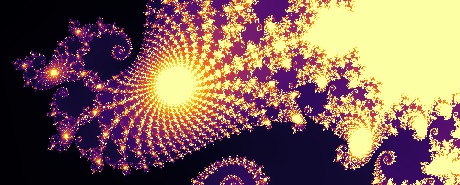Short description Key concepts: mathematics, art Duration: 3 hour
Using beautiful mathematical objects and art to fight math phobia.This is an “exploratory math” outreach activity, specifically aimed at dispelling the thrall of math phobia. There are two parts to that phobia - thinking that math is impossible to learn and that it is dull. Everybody agrees mathematics is useful, but less commonly appreciated, mathematics is something you can learn like anything else (nobody is “just bad at math”, but it seems a lot of people have wrongly internalized that rote memorization is all there is to math). Moreover, something people miss when talking to kids is that mathematics is something that can be pretty. Here we use the prettiness to trick kids into playing with math. We avoid the “scary” algebra by using purely numerical work and a lot of guided exploration. To facilitate the exploration, we use a programming language as a calculating and plotting tool.

The final goal is to have the students be able to plot and explore natural fractals on their own. We will start by presenting a simple language for calculations and plotting (the Julia programming language). We learn the basics of manipulating lists of numbers, repeating operations until a condition is met, and plotting lines with given coordinates through the first hour or so. We introduce the notion of dynamical laws (using the example of population growth and avoiding any mathematical jargon) in the first half of the second hour. We introduce Mandelbrot’s “dynamical law” and then we let the students explore its convergence properties (by giving them the code to do that) for another half an hour. In the third hour we explain and rebuild that code from scratch.

The students will be able to build something similar to the following artwork (present implementation based on work by James Henstridge):

Click on it to zoom to a predetermined point.

All of the code can be ran on any personal computer by installing the open source Julia language or by using a free online platform like Juliabox.com. We provide a number of resources for students and instructors:

• A short notebook that explains how to install the Plotting library in Julia 1.0.
• A detailed notebook with all of the required code and explanations to how it works, mainly useful for self-study or for instructor preparation. This is the main resource! It is quite long, but skimming through it and looking through the visualizations at the very end describes in more detail what the students would be able to create at the end of the activity.
• A "Sing-along" set of notebooks (1 and 2) that can be used to interactively teach the students - the instructor guides them in writting the notebook content together.
• A short "Coding Pedagogy" notebook with an effective way to write (or teach to write) simple computer functions.
• A couple of "Mandelbrot Plotter" (here with custom colors)notebooks with just the code necessary for plotting the Mandelbrot fractals.